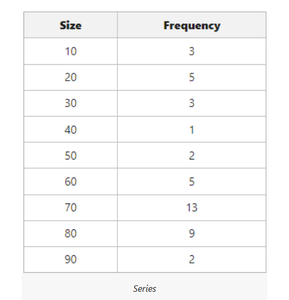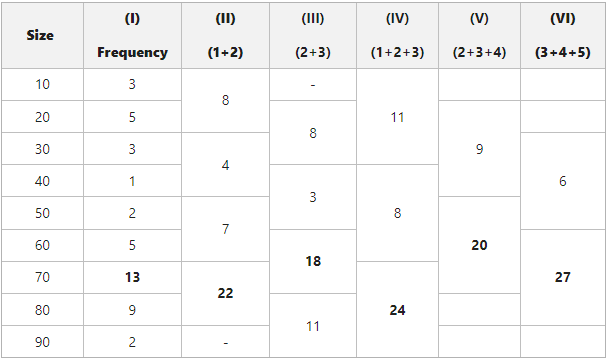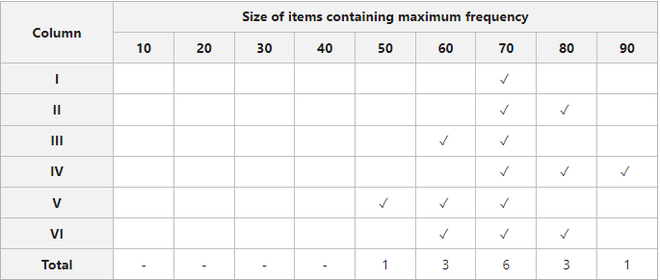GFG App
Open AppBrowser
Continue

# Grouping Method of Calculating Mode in Discrete Series

Mode is the value from a data set that has occurred the most number of times. It is one of the most crucial measures of central tendency and is represented by Z. For example, if, in a class of 35 students, 10 students are 10 years old, 20 students are 11 years old, and the rest 5 students are 9 years old, the modal age of the class will be 11 years. There are two methods of determining mode, one is the inspection method, and the other is the grouping method. Initially, we use the inspection method to calculate mode; however, if it becomes impossible to locate the modal value with the help of the inspection method, we can use the grouping method.

## Grouping Method

It is the method of calculating mode in cases where more than one value has the highest frequency and it becomes impossible to find the mode. The grouping method involves the preparation of two tables; viz., the Grouping Table and the Analysis Table. Here are the steps to prepare these two tables for the calculation of mode.

### Grouping Table

1. First of all, take the frequencies in the first column.
2. Then add the frequencies in two(s) in the second column.
3. In the third column add frequencies in two(s), after leaving the first frequency.
4. The fourth step involves adding frequencies in three(s).
5. In the next step, leave the first frequency and add the rest in three(s) in the fifth column.
6. In the last step of preparing the grouping table, add the frequencies in three(s) after leaving the first two frequencies.

After following the six steps, we will circle or mark the maximum total of each of the columns.

### Analysis Table

1. First of all, note down the highest totals in every column.
2. After that, note down the variable or/and variables in every column that corresponds to that total.
3. In the third step, check if the total in the previous step is of one term or more than one term.
4. If the total in the second step is of more than one term, mark those variables with ✓ or x.
5. Now, count the ✓ or x of each column.

The variable with the maximum number of ✓ or x will be the mode of the series.

#### Example: Find the mode of the following series:Grouping Table

• The first column includes the frequency of the series of data. In this column, we have marked 13 as it is the highest value.
• The second column includes the sum of frequencies in two(s). [3+5 = 8, 3+1 = 4, 2+5 = 7, and 13+9 = 22]. In this column, we have marked 22 as it is the highest value.
• The third column includes the sum of frequencies in two(s) after leaving the first one; i.e., 3. [5+3 = 8, 1+2 = 3, 5+13 = 18, and 9+2 = 11]. In this column, we have marked 18.
• The fourth column includes the sum of frequencies in three(s). [3+5+3 = 11, 1+2+5 = 8, 13+9+2 = 24] In this column, we have marked 24.
• The fifth column includes the sum of frequencies in three(s) after leaving the first one; i.e., 3. [5+3+1 = 9 and 2+5+13 = 20] In this column, we have marked 20.
• The sixth column includes the sum of frequencies in three(s) after leaving the first two; i.e., 3 and 5. [3+1+2 = 6 and 5+13+9 = 27]. In this column, we have marked 27.Analysis Table

• 13 is the highest value in the first column and it is an individual frequency of size 70. Therefore, we have tick marked 70.
• 22 is the highest value in the second column and it is the sum of 13 and 9; i.e., of sizes 70 and 80. Therefore, we have tick-marked both sizes.
• 18 is the highest value in the third column and it is the sum of 5 and 13; i.e, of sizes 60 and 70. Therefore, we have tick-marked both sizes.
• 24 is the highest value in the fourth column and it is the sum of 13, 9, and 2; i.e, of sizes 70, 80, and 90. Therefore, we have tick-marked the three sizes.
• 20 is the highest value in the fifth column and it is the sum of 2, 5, and 13; i.e., of sizes 50, 60, and 70. Therefore, we have tick-marked the three sizes.
• Ultimately, 27 is the highest value of the sixth column and it is the sum of 5, 13, and 9; i.e., of sizes 60, 70, and 80. Therefore, we have tick-marked the three sizes.

#### MODE (Z) = 70

My Personal Notes arrow_drop_up